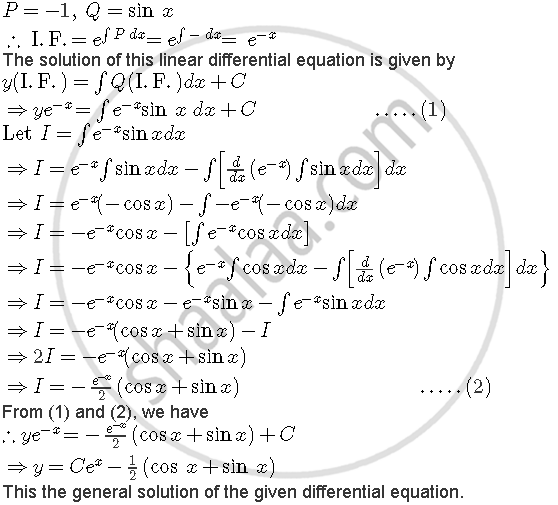Share

# Find the General Solution of the Differential Equation Dybydx -y = Sin X - CBSE (Commerce) Class 12 - Mathematics

ConceptMethods of Solving First Order, First Degree Differential Equations Linear Differential Equations

#### Question

Find the general solution of the differential equation dy/dx - y = sin x

#### Solution

The given differential equation is

dy/dx - y = sin x .....(1)

Clearly, it is a linear differential equation of the form dy/dx + Py=Q

HereIs there an error in this question or solution?

#### Video TutorialsVIEW ALL 

Solution Find the General Solution of the Differential Equation Dybydx -y = Sin X Concept: Methods of Solving First Order, First Degree Differential Equations - Linear Differential Equations.
S